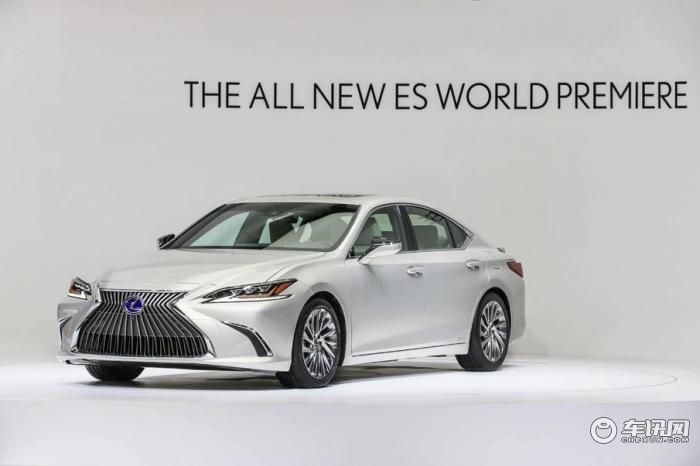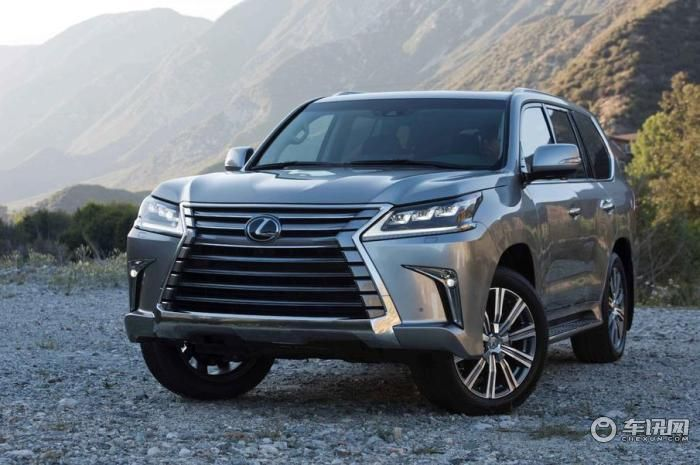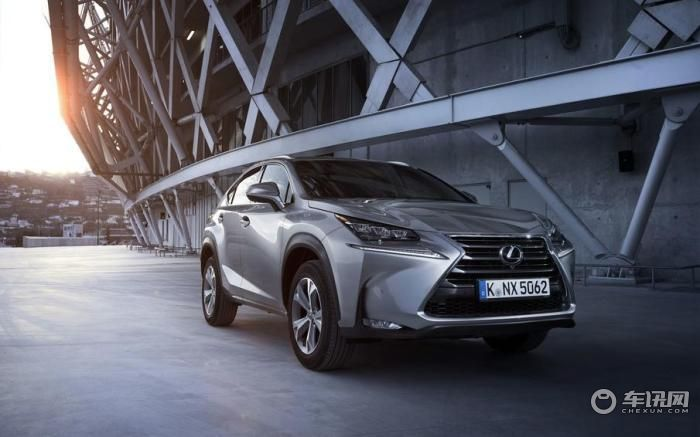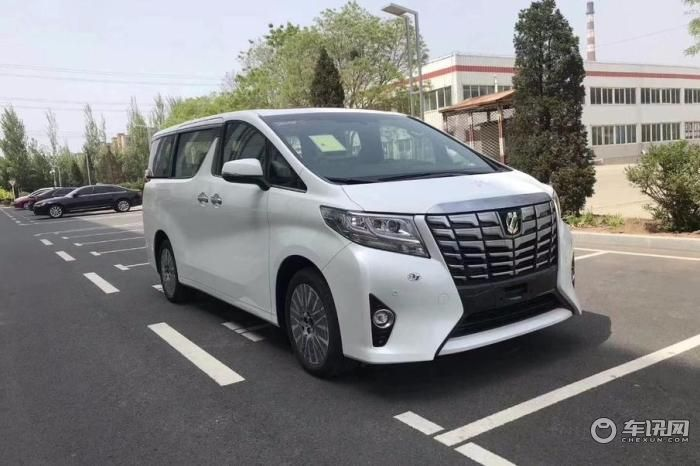## 要么加价，要么等，雷克萨斯为何这么狂？

2019年07月04日 18:24 来源：车行天下 超过：10200次关注

雷克萨斯在中国消费者心目中的地位是越来越高了，曾经他是一个二线豪华品牌，但目前它的销量已经逼近甚至超过国产奥迪了，消费者也一直很期待雷克萨斯什么时候能够国产，能以更便宜的价格买到了雷克萨斯。但是，丰田却一直没有将雷克萨斯国产，而是保持进口销售，所以雷克萨斯在中国市场就成了一个特殊的存在。

在目前中国车市销量不太景气的前提下，各家厂商甚至是豪华品牌都在降价销售，但是唯有雷萨斯不但不降价，而且还加价，如果不加价就需要等待很长的时间，在中国市场是一个很奇怪的现象。雷克萨斯ES系列

最近我们获悉2018款雷克萨斯ES200，卓越版，指导价27.9万元，现在加价1万到2万之间，如果选择不加价，可能需要等1~2个月，甚至是三个月以后提车，而雷克萨斯ES300h2018款卓越版加价2万元上下，雷克萨斯ES260，2018款卓越版加加2.3万元左右。雷克萨斯LX570

2019款 动感豪华车型，指导价129.1万，现在加价17万左右。2019款 尊贵豪华，指导价133.9万，现在加价19万左右。2019款 巅峰特别版，指导价141.7万，现在加价27万左右。雷克萨斯NX

雷克萨斯NX300h 18款，前驱 锋尚版，指导价37.4万，现在加价一万左右。

为什么雷克萨斯车型加价销售依然供不应求呢?其实本质上来说还是因为产品力比较优秀。

产品力优秀可以从两个方面来分析，首先是在设计层面，在全新一代雷克萨斯产品系列刚问世的时候，很多消费者对这种夸张的造型设计不屑一顾。甚至会觉得与主流的设计格格不入。但其实我们可以发现，在年轻消费者崛起之后，他们渴望特立独行，喜欢我行我素的这种风格，与雷克萨斯的设计其实不谋而合。所以雷克萨斯也就成为了目前市面上造型设计最夸张，最犀利的品牌，甚至没有之一了，所以消费者不选雷克萨斯又能选谁呢?

第二个层面，我们可以发现，雷克萨斯并非是同级别同价位车型中最豪华的，也不是同级别同价位车型当中，空间最大的。甚至不是同级别同价位车型当中科技感最强的，但是雷克萨斯却可以把车型制作的特别的省心，而且耐用，给消费者后续的用车带来实惠。这也是其他车型不能比的。物以稀为贵

第三个层面其实是市场供求关系引起的，其实不仅是雷克萨斯这个品牌，而且也包括丰田旗下的部分车型，倒不是由于它多么的豪华，而是由于生产量比较小，所以物以稀为贵，也就让雷克萨斯的汽车变得贵了。

虽然雷克萨斯的加价销售，并非厂商刻意为之，而是经销商所为，但对于品牌形象来说自然是有所损害的。不过雷克萨斯好像并不在意这些。依然我行我素，花更多的精力和时间做好产品，我觉得对于中国汽车品牌来说，也是一个不小的启示。

#### 相关文章

0-500 字已有评论 0条 查看评论>>

### 热门标签

﻿
• 快速找车
• 选择品牌
• 选择品牌
• A  奥迪
• A  阿斯顿·马丁
• A  阿尔法·罗密欧
• B  宝沃
• B  布加迪
• B  巴博斯
• B  保时捷
• B  宾利
• B  奔驰
• B  宝马
• B  本田
• B  别克
• B  标致
• B  比亚迪
• B  宝骏
• B  北汽制造
• B  北汽新能源
• B  北汽幻速
• B  北汽威旺
• B  北京汽车
• B  奔腾
• B  北汽绅宝
• C  长安
• C  长安商用
• C  长城
• C  昌河
• D  大众
• D  道奇
• D  DS
• D  东南
• D  东风风神
• D  东风风行
• D  东风小康
• D  东风风度
• D  东风
• F  福特
• F  丰田
• F  菲亚特
• F  法拉利
• F  福田
• F  福迪
• F  福汽启腾
• G  观致
• G  广汽传祺
• G  广汽吉奥
• G  GMC
• H  红旗
• H  汉腾汽车
• H  哈弗
• H  哈飞
• H  海格
• H  海马
• H  华颂
• H  黄海
• H  华泰
• H  恒天
• J  吉利汽车
• J  捷豹
• J  Jeep
• J  江淮
• J  江铃
• J  金杯
• J  九龙
• J  金旅
• K  凯翼
• K  凯迪拉克
• K  克莱斯勒
• K  科尼塞克
• K  卡威
• K  开瑞
• L  路虎
• L  林肯
• L  劳斯莱斯
• L  兰博基尼
• L  雷克萨斯
• L  铃木
• L  雷诺
• L  理念
• L  力帆
• L  莲花汽车
• L  猎豹
• L  路特斯
• L  陆风
• M  马自达
• M  MG
• M  MINI
• M  玛莎拉蒂
• M  摩根
• M  迈凯轮
• N  纳智捷
• O  欧宝
• O  讴歌
• O  欧朗
• Q  奇瑞
• Q  起亚
• Q  启辰
• R  日产
• R  荣威
• R  瑞麒
• S  三菱
• S  斯威汽车
• S  萨博
• S  smart
• S  斯柯达
• S  斯巴鲁
• S  思铭
• S  双龙
• S  上汽大通
• S  双环
• T  特斯拉
• T  腾势
• W  沃尔沃
• W  五菱汽车
• W  五十铃
• W  威兹曼
• W  威麟
• X  现代
• X  雪佛兰
• X  雪铁龙
• X  西雅特
• Y  一汽
• Y  英菲尼迪
• Y  英致
• Y  依维柯
• Y  野马汽车
• Y  永源
• Z  众泰
• Z  中华
• Z  中兴
• Z  知豆
• 选择车系
• 选择车系
• 车型对比
• 选择品牌
• 选择品牌
• A  奥迪
• A  阿斯顿·马丁
• A  阿尔法·罗密欧
• B  宝沃
• B  布加迪
• B  巴博斯
• B  保时捷
• B  宾利
• B  奔驰
• B  宝马
• B  本田
• B  别克
• B  标致
• B  比亚迪
• B  宝骏
• B  北汽制造
• B  北汽新能源
• B  北汽幻速
• B  北汽威旺
• B  北京汽车
• B  奔腾
• B  北汽绅宝
• C  长安
• C  长安商用
• C  长城
• C  昌河
• D  大众
• D  道奇
• D  DS
• D  东南
• D  东风风神
• D  东风风行
• D  东风小康
• D  东风风度
• D  东风
• F  福特
• F  丰田
• F  菲亚特
• F  法拉利
• F  福田
• F  福迪
• F  福汽启腾
• G  观致
• G  广汽传祺
• G  广汽吉奥
• G  GMC
• H  红旗
• H  汉腾汽车
• H  哈弗
• H  哈飞
• H  海格
• H  海马
• H  华颂
• H  黄海
• H  华泰
• H  恒天
• J  吉利汽车
• J  捷豹
• J  Jeep
• J  江淮
• J  江铃
• J  金杯
• J  九龙
• J  金旅
• K  凯翼
• K  凯迪拉克
• K  克莱斯勒
• K  科尼塞克
• K  卡威
• K  开瑞
• L  路虎
• L  林肯
• L  劳斯莱斯
• L  兰博基尼
• L  雷克萨斯
• L  铃木
• L  雷诺
• L  理念
• L  力帆
• L  莲花汽车
• L  猎豹
• L  路特斯
• L  陆风
• M  马自达
• M  MG
• M  MINI
• M  玛莎拉蒂
• M  摩根
• M  迈凯轮
• N  纳智捷
• O  欧宝
• O  讴歌
• O  欧朗
• Q  奇瑞
• Q  起亚
• Q  启辰
• R  日产
• R  荣威
• R  瑞麒
• S  三菱
• S  斯威汽车
• S  萨博
• S  smart
• S  斯柯达
• S  斯巴鲁
• S  思铭
• S  双龙
• S  上汽大通
• S  双环
• T  特斯拉
• T  腾势
• W  沃尔沃
• W  五菱汽车
• W  五十铃
• W  威兹曼
• W  威麟
• X  现代
• X  雪佛兰
• X  雪铁龙
• X  西雅特
• Y  一汽
• Y  英菲尼迪
• Y  英致
• Y  依维柯
• Y  野马汽车
• Y  永源
• Z  众泰
• Z  中华
• Z  中兴
• Z  知豆
• 选择车系
• 选择车系
• 选择车型
• 选择车型
• 意见反馈